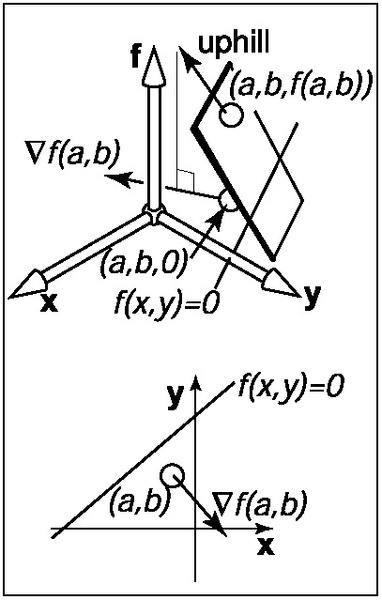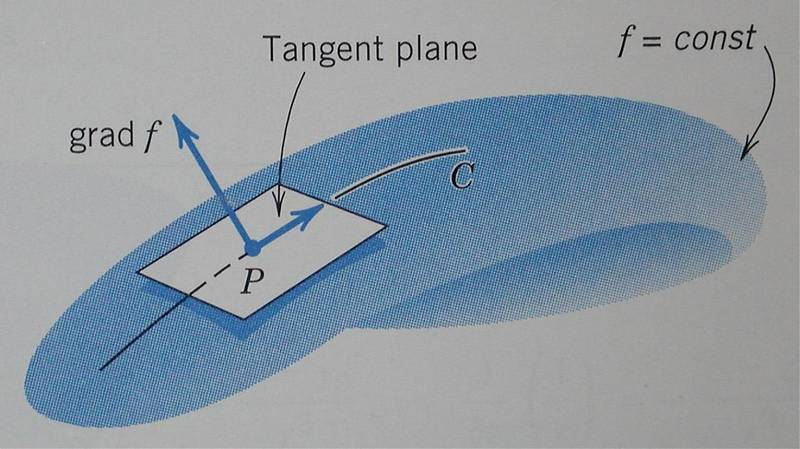In the context of height fields, the geometric meaning of partial derivatives and gradients is more visible than usual. Suppose that near the point (a, b), f(x, y) is a plane (the above figure). There is a specific uphill and downhill direction. At right angles to this direction is a direction that is level with respect to the plane. Any intersection between the plane and the f(x, y) = 0 plane will be in the direction that is level. Thus the uphill/downhill directions will be perpendicular to the line of intersection f(x, y) = 0.

I read a book about gradient vector but I don't understand what the above underlined sentences meant.

Can you tell me what they mean?

Thanks.

Last edited:

If you look at the graphic where f(x, y) is zero, if you draw a perpendicular line from that point, you will see that the gradient is pointing from that direction.

HallsofIvy
Homework Helper
View attachment 14310

In the context of height fields, the geometric meaning of partial derivatives and gradients is more visible than usual. Suppose that near the point (a, b), f(x, y) is a plane (the above figure). There is a specific uphill and downhill direction. At right angles to this direction is a direction that is level with respect to the plane. Any intersection between the plane and the f(x, y) = 0 plane will be in the direction that is level. Thus the uphill/downhill directions will be perpendicular to the line of intersection f(x, y) = 0.

I read a book about gradient vector but I don't understand what the above underlined sentences meant.

Can you tell me what they mean?

Thanks.
First of all, those sentences don't say anything about "partial derivatives" or "gradient". What it does say is that if you have a surface (or plane) z= f(x,y) and intersect it with the xy-plane, z= 0, then z does not change and so it's "height" (z-value) is constant: 0. That is what it means by "level". Of course, the same would be true in the case of any plane of the form z= a where a is a constant.

While the sentences don't say anything about the "gradient" it does appear in the picture. One of the things you can show about the gradient is that the rate of change in any particular direction, say at angle $\theta$ to the x-axis, is $cos(\theta)\partial f/\partial x+ sin(\theta)\partial f/\partial y= (cos(\theta)\vec{i}+ sin(\theta)\vec{j})\cdot \nabla f$. Since a vector of unit length in that direction would be $cos(\theta)\vec{i}+ sin(\theta)\vec{j}$, that says that the rate of change of a function, in the direction of a unit vector $\vec{v}$ is $\vec{v}\cdot\nabla f[itex]. If, in particular, [itex]\vec{v}$ points in the direction in which f is "level"- i.e. doesn't change- then the "rate of change" in that direction is 0: $\vec{v}\cdot\nabla f= 0$ which says that $\vec{i}$ and $\nabla f$ are perpendicular to each other as BryanP says.

But the picture showed only the projection of inclined plane on the horizontal plane.

That gradient is not the normal vector to that inclined plane.

I got confused with the general concept of gradient.

The gradient should be the normal vector of the tangent of the plane.But the picture showed only the projection of inclined plane on the horizontal plane.

That gradient is not the normal vector to that inclined plane.

I got confused with the general concept of gradient.

The gradient should be the normal vector of the tangent of the plane.

View attachment 14318

what do you mean by that?

tiny-tim
Homework Helper
Welcome to PF!

But the picture showed only the projection of inclined plane on the horizontal plane.

That gradient is not the normal vector to that inclined plane.

I got confused with the general concept of gradient.

The gradient should be the normal vector of the tangent of the plane.

View attachment 14318

Hi chrisyuen! Welcome to PF!The f in your second picture is not the same animal as the f of your first picture.

The second-picture-f (I'll call it "F" from now on) is a three-dimensional function, of x y and z, and it defines a surface by F(x,y,x) = constant.

So the F for your plane would be F(x,y,x) = f(x,y) - z;

so your plane is the surface F(x,y,z) = 0.

The gradient of that F is ∇F(x,y,z) = (∂F/∂x, ∂F/∂y,∂F/∂z), = (∂f/∂x, ∂f/∂y,-1), which is the normal to the plane!What I meant was that the gradient vector is not perpendicular to the plane in the first picture, it was only perpendicular to the "intersection of that plane to f=0" but not that plane. And why can you set F(x,y,x) = f(x,y) - z and F(x,y,z) = 0 for the second picture? I understood the calculation but how can you know (∂f/∂x,∂f/∂y,-1) is perpendicular to that surface?

tiny-tim
Homework Helper
I understood the calculation but how can you know (∂f/∂x,∂f/∂y,-1) is perpendicular to that surface?

Hi chrisyuen!Consider any curve, C, along the surface.

Define a parameter t along C.

So a typical point P(t) on C is at (x(t),y(t),z(t)), and the tangent to C at P(t) is in the direction T = (dx/dt,dy/dt,dz/dt).

Since the surface is defined by F(x,y,z) = 0,

that means that F(x(t),y(t),z(t)) = 0, for all t.

So differentiate w.r.t. t: (∂F/∂x)dx/dt + (∂F/∂y)dy/dt + (∂F/∂z)dz/dt = 0,

which is the same as the dot-product:
(∂F/∂x,∂F/∂y,∂F/∂z).(dx/dt,dy/dt,dz/dt) = 0.

In other words: (∂F/∂x,∂F/∂y,∂F/∂z).T = 0,

and so (∂F/∂x,∂F/∂y,∂F/∂z) is perpendicular to the tangents to all curves in the surface through P(t).

So (∂F/∂x,∂F/∂y,∂F/∂z) is the normal at P(t).[And in this case, that's (∂f/∂x, ∂f/∂y,-1)]